# Maximum flow problem

﻿
Maximum flow problem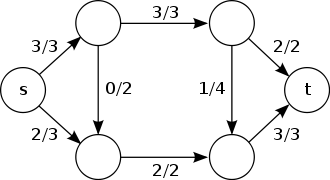An example of a flow network with a maximum flow. The source is s, and the sink t. The numbers denote flow and capacity.

In optimization theory, the maximum flow problem is to find a feasible flow through a single-source, single-sink flow network that is maximum.

The maximum flow problem can be seen as a special case of more complex network flow problems, such as the circulation problem. The maximum value of an s-t flow is equal to the minimum capacity of an s-t cut in the network, as stated in the max-flow min-cut theorem.

## History

The maximum flow problem was first formulated in 1954 by T. E. Harris as a simplified model of Soviet railway traffic flow. In 1955, Lester R. Ford and Delbert R. Fulkerson created the first known algorithm, the Ford–Fulkerson algorithm.

Over the years, various improved solutions to the maximum flow problem were discovered, notably the shortest augmenting path algorithm of Edmonds and Karp and independently Dinitz; the blocking flow algorithm of Dinitz; the push-relabel algorithm of Goldeberg and Tarjan; and the binary blocking flow algorithm of Goldberg and Rao. The electrical flow algorithm of Christiano, Kelner, Madry, and Spielman finds an approximately optimal maximum flow but only works in undirected graphs.

## Definition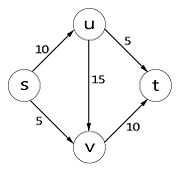A flow network, with source s and sink t. The numbers next to the edge are the capacities.

Let$N = (V, E)$ be a network with$s, t \in V$ being the source and the sink of$N$ respectively.

The capacity of an edge is a mapping$c : E \to \mathbb{R}^+$, denoted by$c_{uv}$ or$c(u, v)$. It represents the maximum amount of flow that can pass through an edge.
A flow is a mapping$f : E \to \mathbb{R}^+$, denoted by$f_{uv}$ or$f(u, v)$, subject to the following two constraints:
1.$f_{uv} \leq c_{uv}$, for each$(u, v) \in E$ (capacity constraint: the flow of an edge cannot exceed its capacity)
2.$\sum_{u:(u, v) \in E} f_{uv} = \sum_{u:(v, u) \in E} f_{vu}$, for each$v \in V \setminus \{s, t\}$ (conservation of flows: the sum of the flows entering a node must equal the sum of the flows exiting a node, except for the source and the sink nodes)
The value of flow is defined by$|f| = \sum_{v \in V} f_{sv}$, where$s$ is the source of$N$. It represents the amount of flow passing from the source to the sink.

The maximum flow problem is to maximize$|f|$, that is, to route as much flow as possible from$s$ to$t$.

## Solutions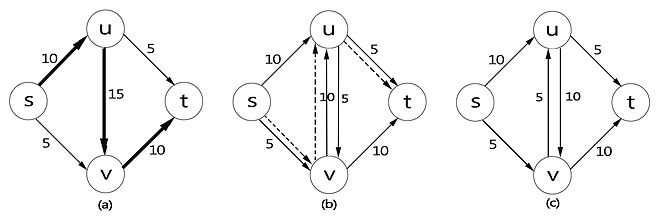(a) The graph G with the path s, u, v, t used to push the first 10 units of flow. (b) The residual graph of the resulting flow f, with the residual capacity next to each edge. The dotted line is the new augmenting path. (c) The residual graph after pushing an additional 5 units of flow along the new augmenting path s, v, u, t.

We can define the Residual Graph, which provides a systematic way to search for forward-backward operations in order to find the maximum flow.

Given a flow network$G$, and a flow$f$ on$G$, we define the residual graph$G_{f}$ of$G$ with respect to$f$ as follows.

1. The node set of$G_{f}$ is the same as that of$G$.

2. Each edge$e = (u, v)$ of$G_{f}$ is with a capacity of$c_{e} - f(e)$.

3. Each edge$e' = (v, u)$ of$G_{f}$ is with a capacity of$f(e)$.

The following table lists algorithms for solving the maximum flow problem.

Method Complexity Description
Linear programming Constraints given by the definition of a legal flow. See the linear program here.
Ford–Fulkerson algorithm O(E max| f |) As long as there is an open path through the residual graph, send the minimum of the residual capacities on the path.

The algorithm works only if all weights are integers. Otherwise it is possible that the Ford–Fulkerson algorithm will not converge to the maximum value.

Edmonds–Karp algorithm O(VE2) A specialization of Ford–Fulkerson, finding augmenting paths with breadth-first search.
Dinitz blocking flow algorithm O(V2E) In each phase the algorithms builds a layered graph with breadth-first search on the residual graph. The maximum flow in a layered graph can be calculated in O(VE) time, and the maximum number of the phases is n-1. In networks with unit capacities, Dinic's algorithm terminates in$\scriptscriptstyle O(V\sqrt{E})$ time.
General push-relabel maximum flow algorithm O(V2E) The push relabel algorithm maintains a preflow, i.e. a flow function with the possibility of excess in the vertices. The algorithm runs while there is a vertex with positive excess, i.e. an active vertex in the graph. The push operation increases the flow on a residual edge, and a height function on the vertices controls which residual edges can be pushed. The height function is changed with a relabel operation. The proper definitions of these operations guarantee that the resulting flow function is a maximum flow.
Push-relabel algorithm with FIFO vertex selection rule O(V3) Push-relabel algorithm variant which always selects the most recently active vertex, and performs push operations until the excess is positive or there are admissible residual edges from this vertex.
Dinitz blocking flow algorithm with dynamic trees O(VE log(V)) The dynamic trees data structure speeds up the maximum flow computation in the layered graph to O(Elog(V)).
Push-relabel algorithm with dynamic trees O(VE log(V2/E)) The algorithm builds limited size trees on the residual graph regarding to height function. These trees provide multilevel push operations.
Binary blocking flow algorithm$\scriptscriptstyle O(E\min(V^{2/3},\sqrt{E})\log(V^2/E)\log{U})$ The value U corresponds to the maximum capacity of the network.
MPM (Malhotra, Pramodh-Kumar and Maheshwari) algorithm O(V3)

For a more extensive list, see .

## Integral flow theorem

The integral flow theorem states that

If each edge in a flow network has integral capacity, then there exists an integral maximal flow.

## Application

### Multi-source multi-sink maximum flow problem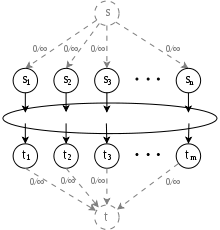Fig. 4.1.1. Transformation of a multi-source multi-sink maximum flow problem into a single-source single-sink maximum flow problem

Given a network N = (V,E) with a set of sources S = {s1, ..., sn} and a set of sinks T = {t1, ..., tm} instead of only one source and one sink, we are to find the maximum flow across N. We can transform the multi-source multi-sink problem into a maximum flow problem by adding a consolidated source connecting to each vertex in S and a consolidated sink connected by each vertex in T with infinite capacity on each edge (See Fig. 4.1.1.).

### Minimum path cover in directed acyclic graph

Given a directed acyclic graph G = (V, E), we are to find the minimum number of paths to cover each vertex in V. We can construct a bipartite graph G' = (VoutVin, E' ) from G, where

1. Vout = {vV: v has positive out-degree}.
2. Vin = {vV: v has positive in-degree}.
3. E' = {(u,v)∈(Vout,Vin): (u,v)∈E}.

Then it can be shown that G' has a matching of size m if and only if there exists n-m paths that cover each vertex in G, where n is the number of vertices in G. Therefore, the problem can be solved by finding the maximum cardinality matching in G' instead.

### Maximum cardinality bipartite matching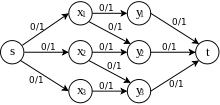Fig. 4.3.1. Transformation of a maximum bipartite matching problem into a maximum flow problem

Given a bipartite graph G = (XY, E), we are to find a maximum cardinality matching in G, that is a matching that contains the largest possible number of edges. This problem can be transformed into a maximum flow problem by constructing a network N = (XY∪{s,t}, E' }, where

1. E' contains the edges in G directed from X to Y.
2. (s,x)∈E' for each xX and (y,t)∈E' for each yY.
3. c(e) = 1 for each eE' (See Fig. 4.3.1).

Then the value of the maximum flow in N is equal to the size of the maximum matching in G.

### Maximum flow problem with vertex capacities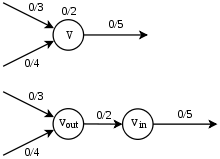Fig. 4.4.1. Transformation of a maximum flow problem with vertex capacities constraint into the original maximum flow problem by node splitting

Given a network N = (V, E), in which there is capacity at each node in addition to edge capacity, that is, a mapping c: VR+, denoted by c(v), such that the flow f has to satisfy not only the capacity constraint and the conservation of flows, but also the vertex capacity constraint

Σi∈Vfivc(v) for each vV∖{s,t}.

In other words, the amount of flow passing through a vertex cannot exceed its capacity.

To find the maximum flow across N, we can transform the problem into the maximum flow problem in the original sense by expanding N. First, each vV is replaced by vin and vout, where vin is connected by edges going into v and vout is connected to edges coming out from v, then assign capacity c(v) to the edge connecting vin and vout (See Fig. 4.4.1). In this expanded network, the vertex capacity constraint is removed and therefore the problem can be treated as the original maximum flow problem.

### Maximum independent path

Given a directed graph G = (V, E) and two vertices s and t, we are to find the maximum number of independent paths from s to t. Two paths are said to be independent if they do not have a vertex in common apart from s and t. We can construct a network N = (V, E) from G with vertex capacities, where

1. s and t are the source and the sink of N respectively.
2. c(v) = 1 for each vV.
3. c(e) = 1 for each eE.

Then the value of the maximum flow is equal to the maximum number of independent paths from s to t.

### Maximum edge-disjoint path

Given a directed graph G = (V, E) and two vertices s and t, we are to find the maximum number of edge-disjoint paths from s to t. This problem can be transformed to a maximum flow problem by constructing a network N = (V, E) from G with s and t being the source and the sink of N respectively and assign each edge with unit capacity.

Wikimedia Foundation. 2010.

### Look at other dictionaries:

• Minimum-cost flow problem — The minimum cost flow problem is finding the cheapest possible way of sending a certain amount of flow through a flow network. Contents 1 Definition 2 Relation to other problems 3 Solutions 4 See also …   Wikipedia

• Minimum cost flow problem — The minimum cost flow problem is finding the cheapest possible way of sending a certain amount of flow through a flow network. Definition Given a flow network ,G(V,E) with source s in V and sink t in V, where edge (u,v) in E has capacity ,c(u,v) …   Wikipedia

• Push-relabel maximum flow algorithm — The push relabel algorithm is one of the most efficient algorithms to compute a maximum flow. The general algorithm has O(V^2 E) time complexity, while the implementation with FIFO vertex selection rule has O(V^3) running time, the highest active …   Wikipedia

• Multi-commodity flow problem — The multi commodity flow problem is a network flow problem with multiple commodities (or goods) flowing through the network, with different source and sink nodes. Contents 1 Definition 2 Relation to other problems 3 Usage 4 …   Wikipedia

• Flow network — In graph theory, a flow network is a directed graph where each edge has a capacity and each edge receives a flow. The amount of flow on an edge cannot exceed the capacity of the edge. Often in Operations Research, a directed graph is called a… …   Wikipedia

• Maximum sustainable yield — In population ecology and economics, maximum sustainable yield or MSY is, theoretically, the largest yield (or catch) that can be taken from a species stock over an indefinite period. Fundamental to the notion of sustainable harvest, the concept… …   Wikipedia

• Max-flow min-cut theorem — In optimization theory, the max flow min cut theorem states that in a flow network, the maximum amount of flow passing from the source to the sink is equal to the minimum capacity which when removed in a specific way from the network causes the… …   Wikipedia

• Closure problem — A Closure problem is a problem in graph theory for finding a set of vertices in a directed graph such that there are no edges from the set to the rest of the graph. More specifically, the minimum closure problem asks for a set of this type with… …   Wikipedia

• Circulation problem — The circulation problem and its variants is a generalisation of network flow problems, with the added constraint of a lower bound on edge flows, and with flow conservation also being required for the source and sink (i.e. there are no special… …   Wikipedia

• Probleme de flot maximum — Problème de flot maximum Un exemple de graphe de flot avec un flot maximum. la source est s, et le puits t. Les nombres indiquent le flot et la capacite. Le problème de flot maximum consiste a trouver un flot réalisable depuis une source unique… …   Wikipédia en Français